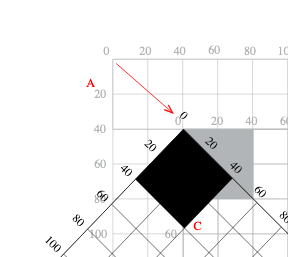# Why do I have to apply matrices in the reverse order

Hi,
I don’t know why but in Glyphs I have to apply matrices in the reverse order.
In order words, In the following code I have to read the last 3 lines from the bottom to the top in order to make some sense of what is going on.

``````newComp = GSComponent( componentName, NSPoint( 0, 0) )

newComp.applyTransform( make_scale_matrix(random.gauss(0.1, 0.3)) )
newComp.applyTransform( make_translation_matrix(thisPoint.x, thisPoint.y) )
``````

This is really different from what I’m used to in processing / openframeworks or other frameworks I have used.

Why is this?

Not sure I understand. Why do you apply transfromations in reverse order in the first place?

If I would want this:I would normally first translate 40, 40 and then rotate 45 degrees.
With Glyphs I have to rotate first 45 degrees and then translate 40, 40.

This is how transformations matrixes work. The rotation is always around the origin of the grid, so if you translate first and then rotate, it will also move the object (in this case to 0, -56).

In the other apps it might be that you apply the transformation to the object directly, not to the matrix.

But in this case I would not use the transformation but the `position`, `scale` and and `rotation` properties of the component (when I understand your code).

``````newComp = GSComponent(componentName, NSPoint( 0, 0))
scale = random.gauss(0.1, 0.3)
newComp.scale = (scale, scale)
newComp.rotation = angleRadians * 180 / math.pi # rotation is in Degrees
newComp.position = thisPoint
``````

No, because rotation is always around 0,0. If you want to rotate around something else, you need to translate it first.